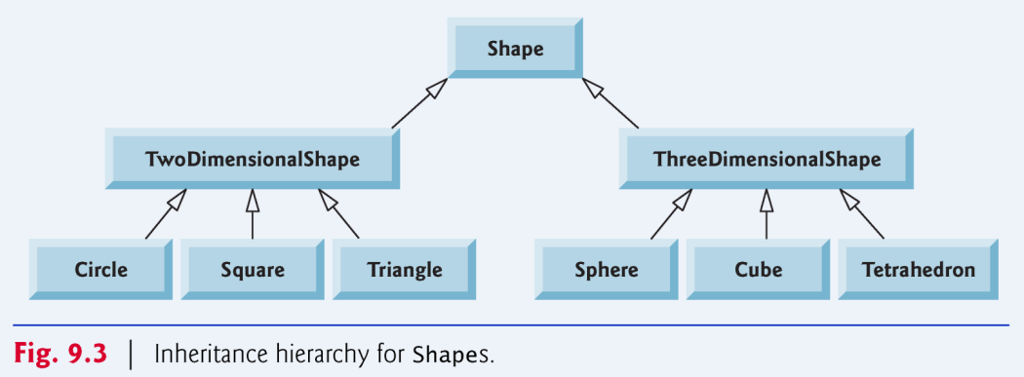# Answered! Implement a Shape hierarchy as shown in the figure above. Each Two Dimensional Shape should contain method…Implement a Shape hierarchy as shown in the figure above. Each Two Dimensional Shape should contain method get Area to calculate the area of the two-dimensional shape. Each Three Dimensional Shape should have methods get Area and get Volume to calculate the surface area and volume, respectively, of the three-dimensional shape. Create a program that uses an array of Shape references to objects of each concrete class in the hierarchy. The program should print a text description of the object to which each array element refers. Also in the loop that processes all shapes in the array, determine whether each shape is a TwoDimensionalShape or a ThreeDimensionalShape. If it is a TwoDimensionalShape, display its area. If it’s a ThreeDimensionalShape, display its area and volume. RUBRIC:

TASK 1: Implement abstract superclass Shape.

Don't use plagiarized sources. Get Your Custom Essay on
Answered! Implement a Shape hierarchy as shown in the figure above. Each Two Dimensional Shape should contain method…
GET AN ESSAY WRITTEN FOR YOU FROM AS LOW AS \$13/PAGE

TASK 2: Implement abstract subclasses TwoDimensionalShape and ThreeDimensionalShape which extend abstract superclass Shape. TwoDimensionalShape contains method getArea, and ThreeDimensionalShape contains methods getArea and getVolume

TASK 3: Implement concrete classes Circle, Square, Triangle, Sphere, Cube and Tetrahedron.

TASK 4: Create a program that uses an array of Shape references to objects of each concrete class in the hierarchy. The loop that processes all shapes in the array determines whether each shape is a Two Dimensional Shape or a Three Dimensional Shape. If it is a Two Dimensional Shape, its area is displayed. If it’s a Three Dimensional Shape, both its area and volume are displayed.

#include <iostream>
#include <cmath>
#include <stdexcept>
#include <memory>
using namespace std;

/**
* Super class
*/
class Shape
{
protected:
int _dimensions;
public:
Shape() : _dimensions{0}
{};
Shape(int dims) : _dimensions{dims}
{};

virtual double getArea() {};
// Because some shapes have no volume.
virtual double getVolume() {};

void setDimensions(int dim)
{
this->_dimensions = dim;
};
int getDimensions()
{
return this->_dimensions;
};
};

/**
* Extended classes
*/
class TwoDimensionalShape : public Shape
{
public:
TwoDimensionalShape() : Shape{2}
{};
// This should throw an error
double getVolume() {
throw logic_error(“This shape ain’t got area!”);
};
};

class ThreeDimensionalShape : public Shape
{
public:
ThreeDimensionalShape() : Shape{3} {};
};

/**
* Final Concrete classes extending extended classes
*/
class Circle : public TwoDimensionalShape
{
protected:
public:
{};
double getArea()
{
// pi*r^2
}
};

class Square : public TwoDimensionalShape
{
protected:
double _side;
public:
Square(double s) : _side{s}
{}
double getArea()
{
// s^2
return pow(_side, 2);
}
};

class Triangle : public TwoDimensionalShape
{
protected:
double _base, _height;
public:
Triangle(double b, double h) : _base{b}, _height{h}
{};
double getArea()
{
// b*h/2
return _base * _height / 2;
}
};

class Sphere : public ThreeDimensionalShape
{
protected:
public:
{}
double getArea()
{
cout << 4 * M_PI * pow(_radius, 2) << endl;
return 4 * M_PI * pow(_radius, 2);
}
double getVolume()
{
return (4/3) * M_PI * pow(_radius, 3);
}
};

class Cube : public ThreeDimensionalShape
{
protected:
double _side;
public:
Cube(double s) : _side{s}
{};
double getArea()
{
// surface area = 6*a^2
return 6 * pow(_side, 2);
}
double getVolume()
{
// a^3
return pow(_side, 3);
}
};

class Tetrahedron : public ThreeDimensionalShape
{
protected:
double _side;
public:
Tetrahedron(double s) : _side{s}
{};
double getArea()
{
// sqrt(3)*a^2
return sqrt(3) * pow(_side, 2);
}
double getVolume()
{
// a^3/6sqrt(2)
return pow(_side, 3) / (6 * sqrt(2));
}
};

int main()
{
unique_ptr<Shape> arr;
arr = make_unique<Circle>(10);
arr = make_unique<Sphere>(10);

// This one is accessing the right method.
cout << “Area of circle: ” << arr->getArea() << endl;
// This one should access the parent, but accesses the grand parent!
// even if it is overridden in parent.
cout << “Volume of circle: ” << arr->getVolume() << endl;

// Both of these are accessing methods on grand parent rather than their own!!
cout << “Area of sphere: ” << arr->getArea() << endl;
cout << “Volume of sphere: ” << arr->getVolume() << endl;

return 0;
}